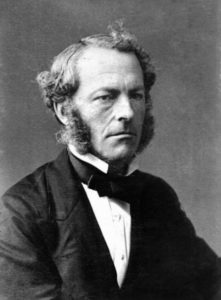## Introduction

When you play in a river you are surrounded by fluids, including water and air. At first it might seem strange to think of air as a fluid, but a fluid is defined as a substance that flows. Wind, therefore, is a great example of air that flows. Other examples of flows include traffic patterns and electrical currents. Flows can be turbulent like what you may experience in airplanes.

Early in the 19th Century, Claude-Louis Navier in France and George Gabriel Stokes in England both derived an equation that can explain and predict the flow of fluids. The Navier-Stokes equations are a system of equations used to describe the velocity of a fluid as it moves through three-dimensional space over a specific interval of time.Sir George Gabriel Stokes

Interestingly, our understanding of solutions to the Navier-Stokes equations remains minimal. Surprisingly, given the equations’ wide range of practical uses, it has not yet been proven that solutions always exist in three dimensions. The Clay Mathematics Institute has called this one of the seven most important open problems in mathematics and has offered a US \$1,000,000 prize for a solution or a counter-example.

In this section, we will learn how to graph systems of equations in two dimensions and find whether solutions exist. We will also see how systems of equations can be used to solve problems where we have two unknown variables.